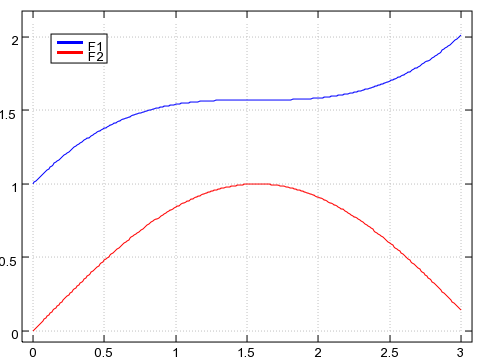# The choice - 2 (J and LISP)

2011 Jul 21

[ Get the code at github ]

We’re using RK4 to solve:

\begin{aligned} \frac{df_1(x)}{dx} =& 1 - f_2(x)\ & \ \frac{df_2(x)}{dx} =& f_1(x) - x \end{aligned}

with initial conditions:

\begin{aligned} x_0 &= 0\ f_1(x_0) &= 1\ f_2(x_0) &= 0 \end{aligned}

in the range $$[0,3]$$.

See the introductory post for details.

As already noted, the code below will be rather idiomatic for each language.

## J

[ J is a terse array programming language. It’s on GPLv3 since March 2011 ]

Resisting the temptation to reduce the code to a line of illegible, swear-like bunch of characters, here is the full code in just 8 crystal clear lines.

<{{static/src/the-choice-2-j-and-lisp/rk4.ijs}}[1,15]

Extendable, expandable, short.

For example:

<{{static/src/the-choice-2-j-and-lisp/rk4.ijs}}[16,]

That gives us the vector v with all the value-pairs $$(x,y1,y2)$$

v
0       1          0
0.01 1.00995 0.00999983
0.02  1.0198  0.0199987
0.03 1.02955  0.0299955
0.04  1.0392  0.0399893
0.05 1.04875  0.0499792
...
2.97 1.98469   0.170752
2.98 1.99303    0.16089
2.99 2.00147   0.151013
3 2.01001    0.14112


which we can conveniently plot:

load 'plot'
'key f1, f2' plot ({. ; (1 { ]) ,: 2 { ]) |: v## LISP

<{{static/src/the-choice-2-j-and-lisp/rk4.cl}}[,16]

For example:

<{{static/src/the-choice-2-j-and-lisp/rk4.cl}}[18,29]

which is indeed the same solution as before:

<{{static/src/the-choice-2-j-and-lisp/rk4.cl}}[31,]

Coming up: R, Fortran, Python, Haskell, Pari/GP, maxima.

Any other languages that you would like to see?